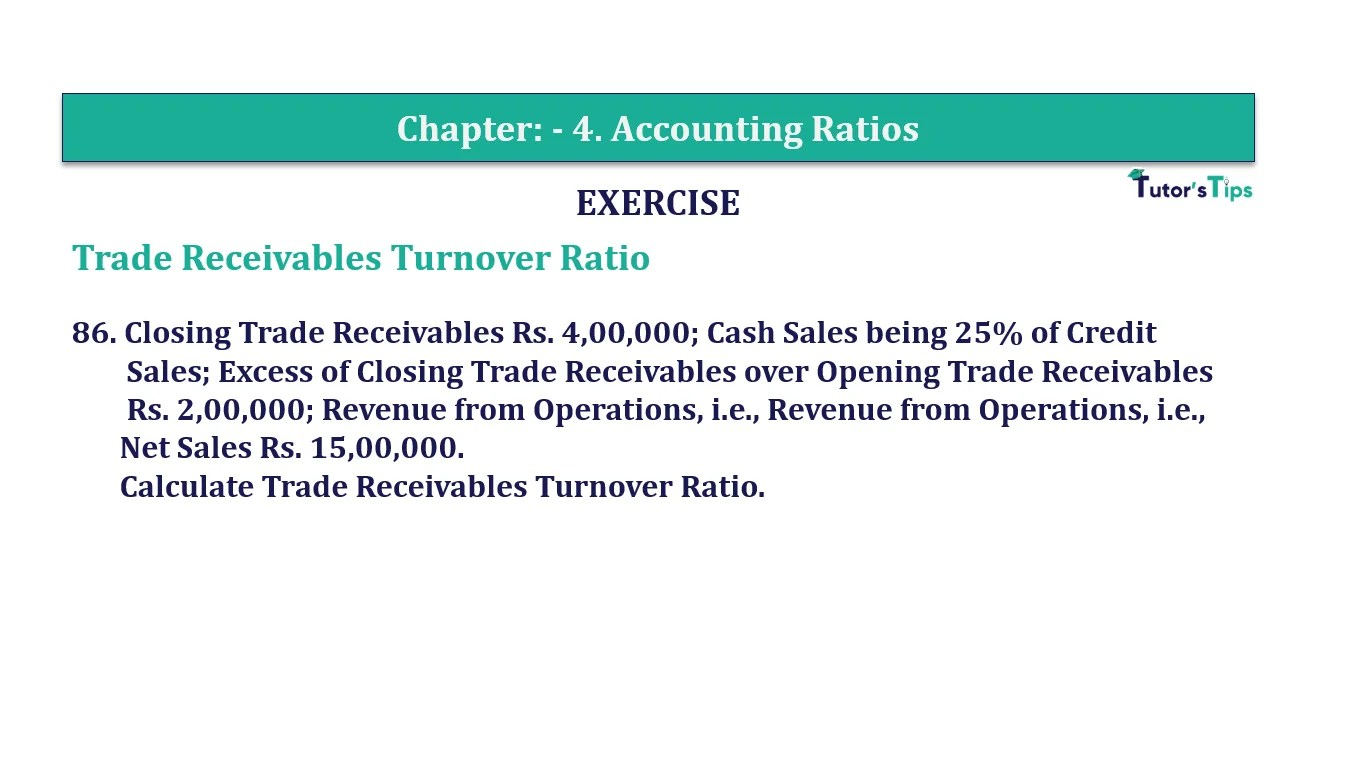# Question 86 Chapter 4 of +2-B – T.S. Grewal 12 ClassQuestion No. 86- Chapter No.4 - T.S. Grewal +2 Book Part B

Question 86 Chapter 4 of +2-B

86. Closing Trade Receivables Rs. 4,00,000; Cash Sales being 25% of Credit
Rs. 2,00,000; Revenue from Operations, i.e., Revenue from Operations, i.e., Net Sales Rs. 15,00,000.
Calculate the Trade Receivables Turnover Ratio.

### The solution of Question 86 Chapter 4 of +2-B: –

 Credit Sales = X Cash Sales = 25% of Credit Sales Cash Sales = X x 25 100 25x 100 Total Sales = Cash Sales + Credit Sales Rs. 15,00,000 = X + 25 100 Rs. 15,00,00,000 = 125x

 X = Rs. 15,00,00,000 25 = 12,00,000

 Opening Trade Receivables = Closing Trade Receivables − 2,00,000 = Rs. 4,00,000 − Rs. 2,00,000 = Rs. 2,00,000

 = Rs. 2,00,000 + Rs. 4,00,000 2 = Rs. 3,00,000

 = Rs. 12,00,000 Rs. 3,00,000 Trade Receivable Turnover Ratio = 4 Times

Balance Sheet: Meaning, Format & Examples

Comment if you have any question.

Also, Check out the solved question of previous Chapters: –